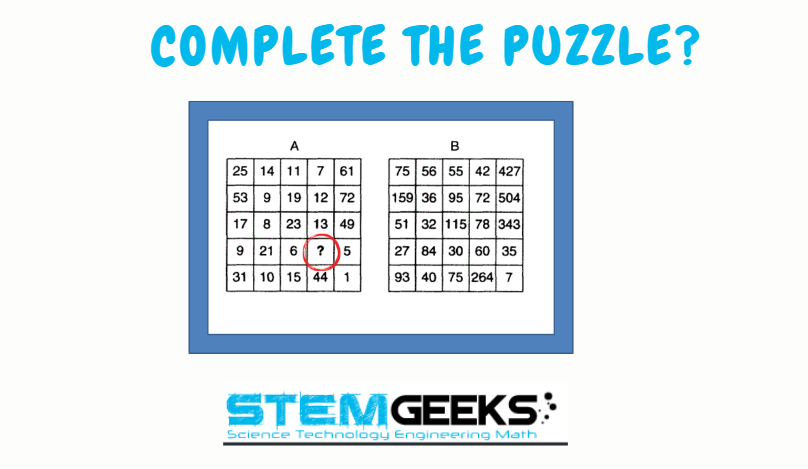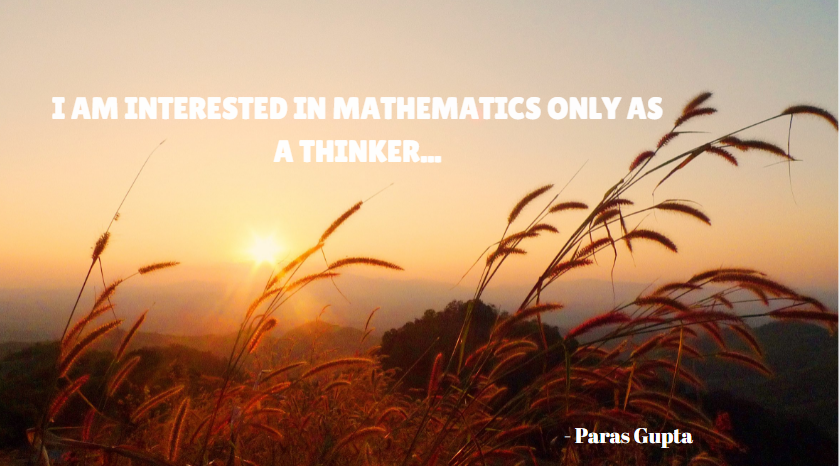# Maths Brain Teasers 85 :: Can You Complete The Puzzle?

in STEMGeeks2 years ago

Hey All; @StemGeeks Mathematician;

Without further, ado, let's get to our puzzle for today:: Maths Brain Teasers 85 :: Can You Complete The Puzzle?Have a close look at the image given and see if you can decode the logic given in the image to complete the puzzle. Try finding out the right NUMBER that should replace the question mark? There is logic to resolve the puzzle; try finding out the logic and it should be resolved in seconds.. Guesswork isn't going to help...

With that, I'll leave you all with the Maths Brain Teasers 85 :: Can You Complete The Puzzle? Good luck solving the puzzle...

##### Maths Brain Teasers 84:: Can you Find the Missing Number? - Solved with ExplanationLet's have a look at the Step by Step instructions to Solve the Maths Brain Teasers 84:: Can you Find the Missing Number?

If you carefully look at the Image in question; You would have noticed the following pattern is being followed::

### 71 (9) 79

• First Number from digit 71 - 7 * Second Number from digit 79 - 9

### 7 * 9 = 63

• Second Number digit from 71 - 1 * First Number digit from 79 - 7

### 71 (9) 79

In the similar fashion lets take the row in question::

### (8 * 9) (6) (2* 6)

Hence the answer to the Maths Brain Teasers 84:: Can you Find the Missing Number? is :: 6

### STEM token GiveAway

I'll be again doing a giveaway of STEM tokens to the lucky random winner with the correct answer. For the last contest, which was Maths Brain Teasers 84:: Can you Find the Missing Number?.

We had 5 entries and I happy to see the engagement by everyone. At the same time, it's great to see the detailed answers provided by everyone as to how they arrived at the solution of the puzzle.. Well done guys.. Way to GO... Now the results part; no entry provided the right answer. So, we don't have a winner for this contest i.e. Maths Brain Teasers 84:: No Winner this Time

### Math Quote for the Day::

Here is the motivation to solve the Maths Equation Puzzle?If you like my work, then please spread the Word.. that we do have the Math Brain Teasers competition here @StemGeeks platform. Reblog is much appreciated.

Best RegardsFind Me on the Other Social Media Platforms::PS:: All the Maths Brain Teasers; are made by me using the Pro Canva License Version

Sort:

The answer is 10....I have a feeling you will get many right answers for this one!

PS Can you show us a solution for the triangle puzzle I still can't get 28 and its beginning to affect my mental health!

Reason

All number in B is the multiple of 2 , 3 , 4, 5 , 6 & 7 of symmetrical A number

9 x 3 = 27
21 x 4 = 84
6 x 5 = 30
? x ? = 60 ? x 6 = 60 = ? = 60/6 = 10
5 x 7 = 35

10 is the answer. Pretty easy one after a difficult brain teaser 84.

A bit late but the answer is 10. I think the other comments pretty much showed how to get the answer.

10!

it's times 3,4,5,6!,7

6*10 = the 60 shown in B

Posted via proofofbrain.io